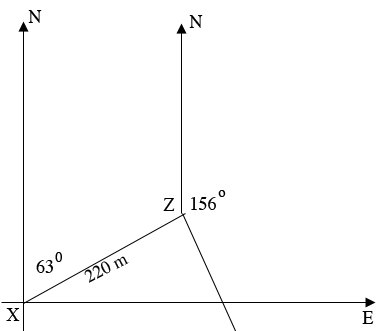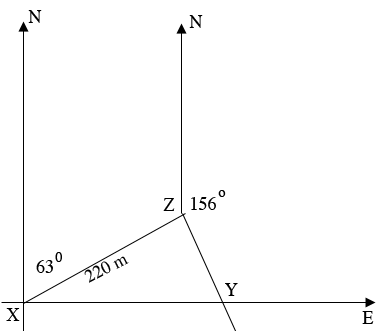SEARCH HOMEMath Central Quandaries & QueriesQuestion from bahlqees: A girl starts from a point X and walks 220m on a bearing 063°.she then walks to a point Y on a bearing 156°.if Y is due east of X,calculate|XY| solve and draw the diagram pleaseHi,

I am going to draw a diagram since I think that is the key to solving this problem. Keep in mind that the bearing of $P$ to $Q$ is the measure of the angle from north to the line segment Joining $P$ to $Q$ measured clockwise from north. Hence I am going to first draw a north south line and an east west line and putting $X$ at their intersection.From $X$ draw a line at a bearing of $63^o$ and place $Z$ on that line and label the distance from $X$ to $Y$ as 220 m. From $Z$ draw a line at bearing $156^{o}.$ The point Z is somewhere ion this line, in fact you know it is due east of $x.$You have enough information now to determine the angle measurements of the interior angles of triangle $XZY.$

Can you now find $|XY|?$

Write back if you need more assistance,

HarleyMath Central is supported by the University of Regina and The Pacific Institute for the Mathematical Sciences.